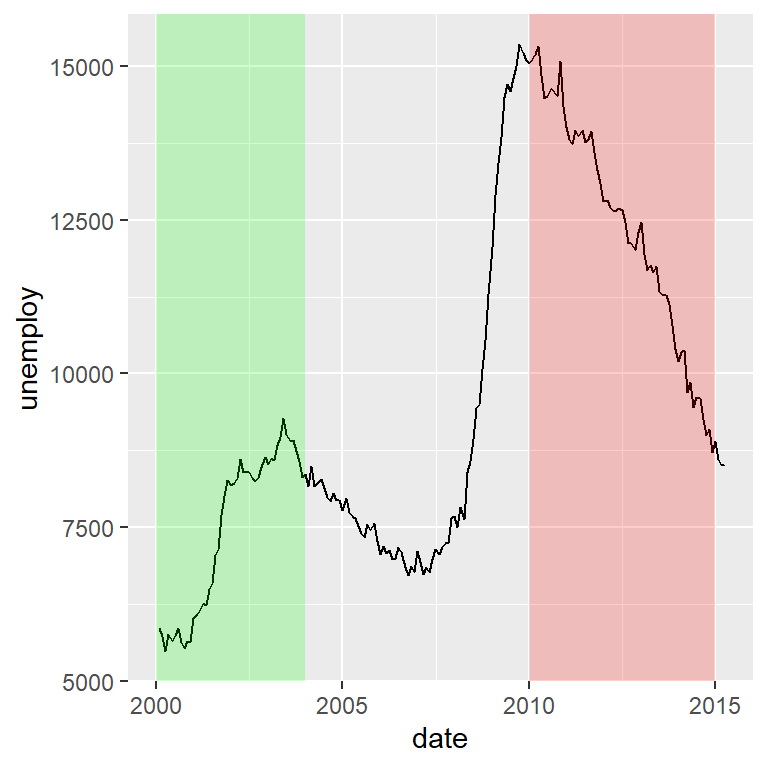# Time series plot in ggplot2

## Plot one or several time series

A time series is the visual representation of time-dependent data, this is, its a chart that represents the evolution of a variable through time. Generally, the pair of points are connected with lines and the decision of showing the points or not depends on your own and also based on the discretization level.

In order to plot a time series in ggplot2 of a single variable you just need a data frame containing dates and the corresponding values of the variable. Note that the date column must be in date format. You will need to input your data and use `geom_line` or `geom_point`.

Time series (line)

``````# install.packages("ggplot2")
library(ggplot2)

# Data with dates and variables. The column 'date' is of class "Date"
df <- economics[economics\$date > as.Date("2000-01-01"), ]

ggplot(df, aes(x = date, y = unemploy)) +
geom_line()``````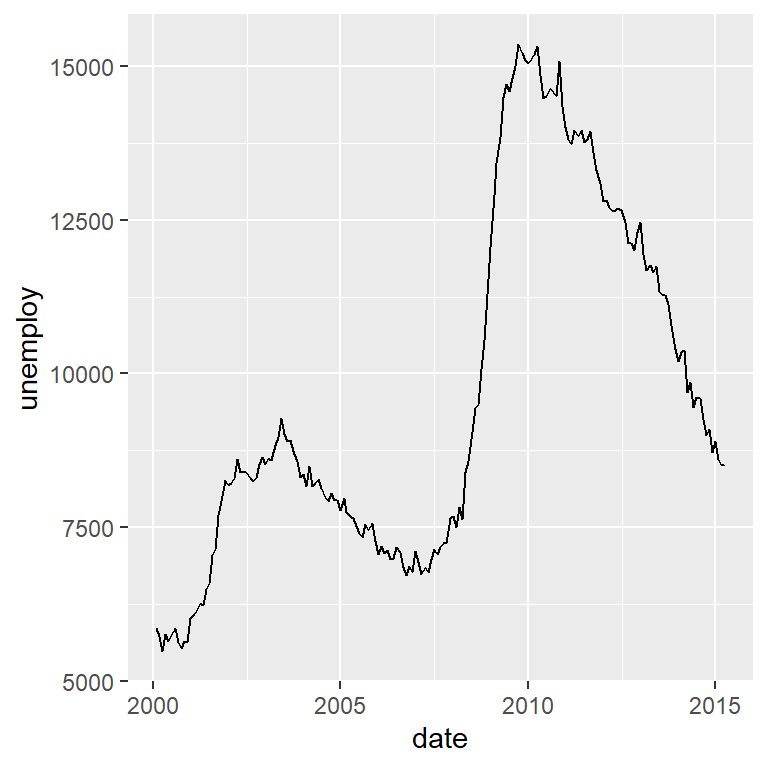As stated before, you can add the points to the time series chart by using the `geom_point` function. In this example there are too many points, making the plot less readable, so we are not going to display them in the following examples.

Time series (line and points)

``````# install.packages("ggplot2")
library(ggplot2)

# Data
df <- economics[economics\$date > as.Date("2000-01-01"), ]

ggplot(df, aes(x = date, y = unemploy)) +
geom_line() +
geom_point()``````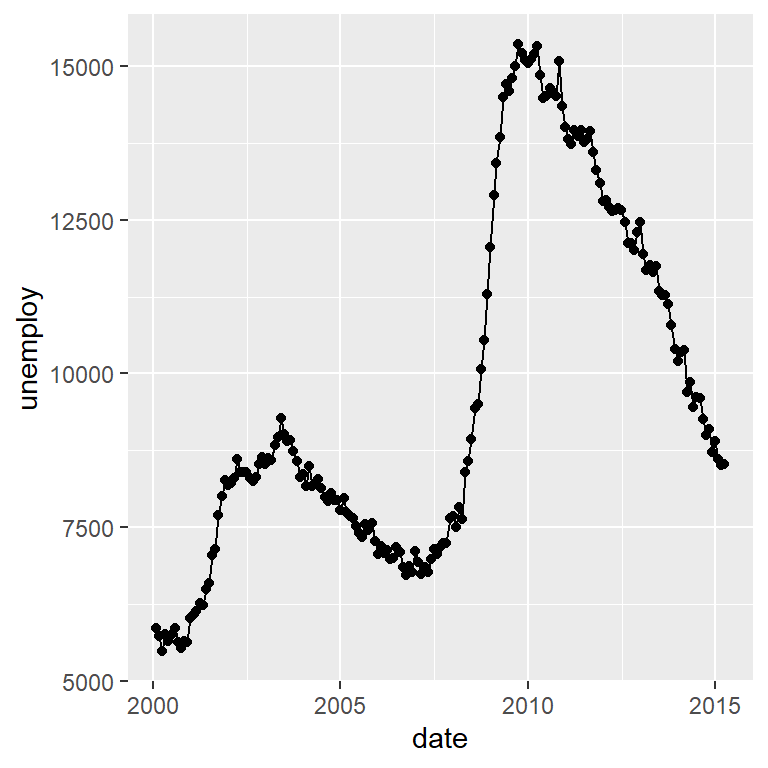The variable passed to `x` inside `aes` must be in a data format. Check the class of the variable with `class(df\$date)` or with `str(df)`. You can use `as.Date` and `as.POSIXct` functions to transform dates into date format if needed.

Time series area

Sometimes time series are plotted with a shaded area. You can add it to your visualization making use of the `geom_area` function.

``````# install.packages("ggplot2")
library(ggplot2)

# Data
df <- economics[economics\$date > as.Date("2000-01-01"), ]

ggplot(df, aes(x = date, y = unemploy)) +
geom_area(fill = "gray", alpha = 0.9) +
geom_line()``````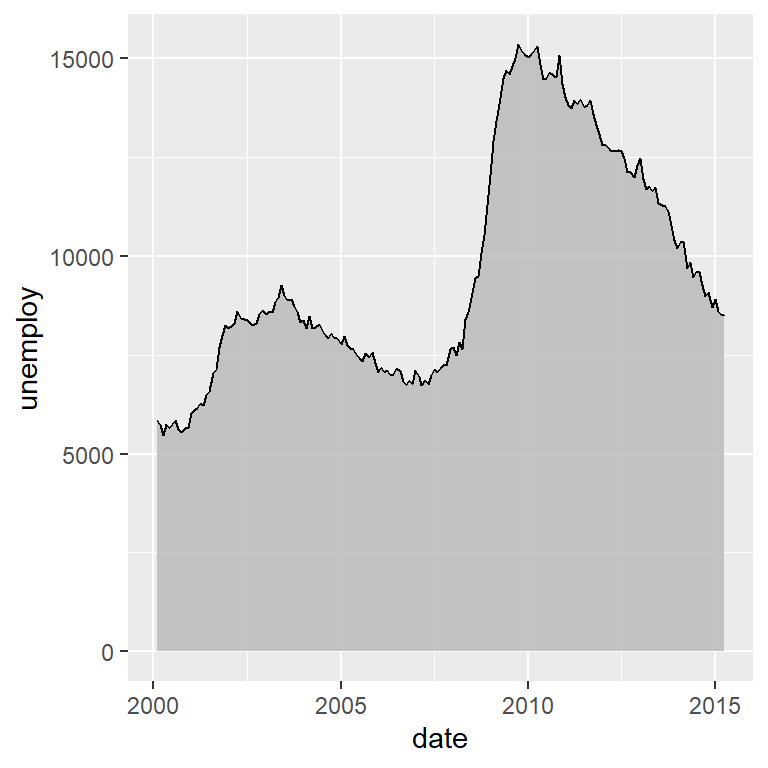Smoothed time series with `stat_smooth`

With `geom_smooth` you can smooth the time series, which can be interesting to analyze trends or patterns. By default, `geom_smooth` will add a confidence interval of the smoothed line, but you can remove it by setting `se = FALSE`.

``````# install.packages("ggplot2")
library(ggplot2)

# Data
df <- economics[economics\$date > as.Date("2000-01-01"), ]

ggplot(df, aes(x = date, y = unemploy)) +
geom_line() +
geom_smooth(se = FALSE)``````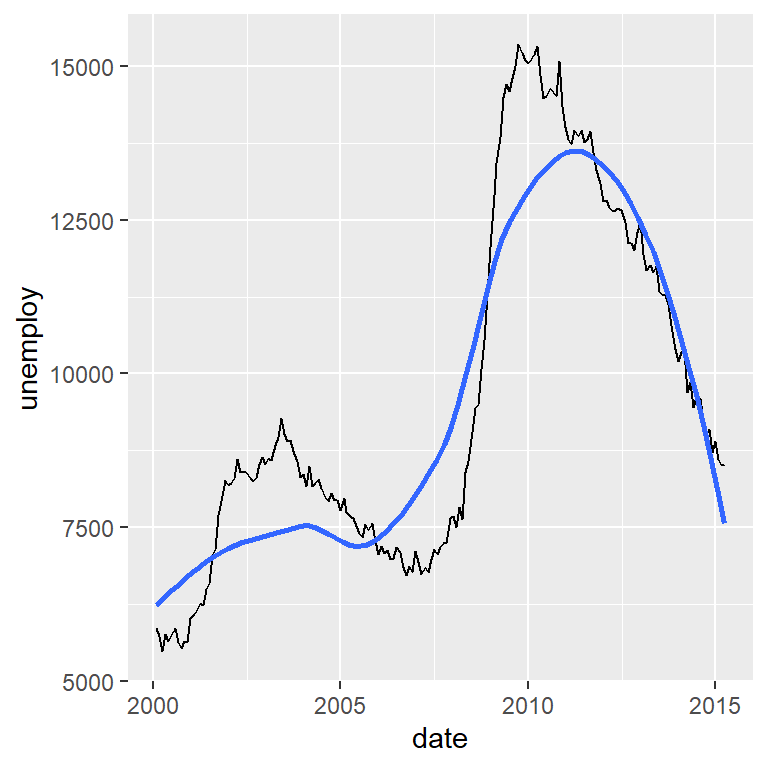Plotting multiple time series on the same graph

In order to plot several time series at once you will need to have your data frame in long format. For this example we will use a subset of the `economics_long` ggplot2 sample data frame.

``````# install.packages("ggplot2")
library(ggplot2)
library(dplyr)

# Data
df2 <- economics_long[economics_long\$date > as.Date("2000-01-01"), ] %>%
filter(variable == "pce" | variable == "unemploy")

ggplot(df2, aes(x = date, y = value, color = variable)) +
geom_line() +
theme(legend.position = "bottom")``````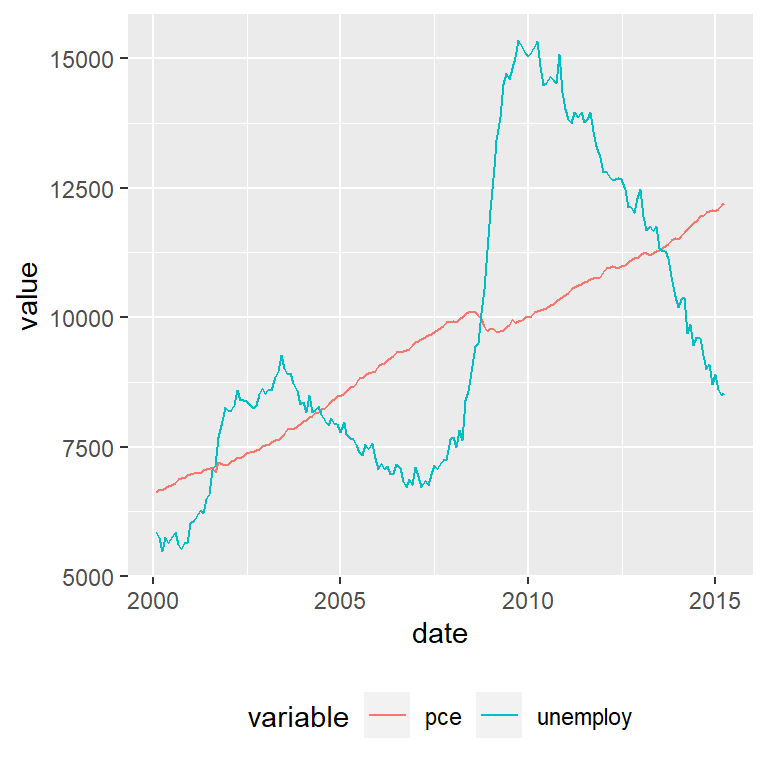The color for each line can be customized with `scale_color_manual`, specifying as many colors as lines with the `values` argument or making used of other discrete color scale function.

Split the time series in several plots by day, week, month, year, …

Making use of ggplot2 facets you can split your time series on predefined intervals. In the following example we are using the `year` function from `lubridate` to extract the corresponding year for each observation and use the new column to split the time series. We set `scales = "free"` to let the axis scales free.

``````# install.packages("ggplot2")
# install.packages("lubridate")
library(ggplot2)
library(lubridate)

# Data
df <- economics[economics\$date > as.Date("2000-01-01"), ]

# New column with the corresponding year for each date
df\$year <- year(df\$date)

ggplot(df, aes(x = date, y = unemploy)) +
geom_line() +
facet_wrap(~year, scales = "free")``````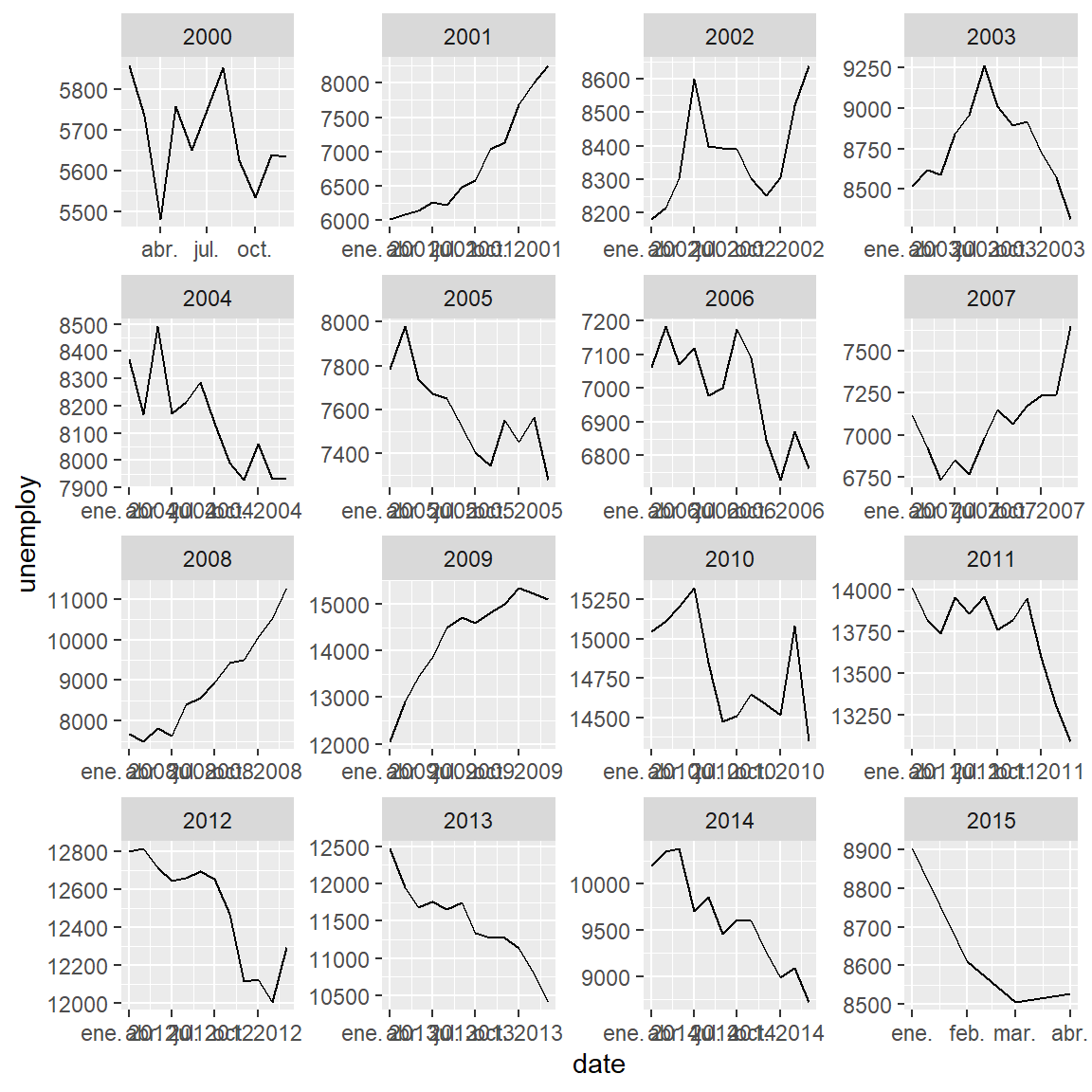## Date format of a time series plot with `scale_x_date`

With the `scale_x_date` function you can customize the X-axis scale when its a date. For instance, you will be able to transform the format of the dates, the limits of the plot or the number of breaks and minor breaks of the axis. In the following table you will see a list of common symbols used to specify different date formats. Note that you can combine the symbols the way you want, as in the examples below.

Symbol Meaning Example
%Y 4 digits year 2023
%y 2 digits year 23
%B Full month name September
%b Abbreviated month name Sep
%m Month as number 09
%d Day of the month 05
%H Hour (00-23) 14
%I Hour (01-12) 14
%M Minute as number 30
%S Second as integer 15
%A Full weekday name Tuesday
%a Abbreviated weekday name Tue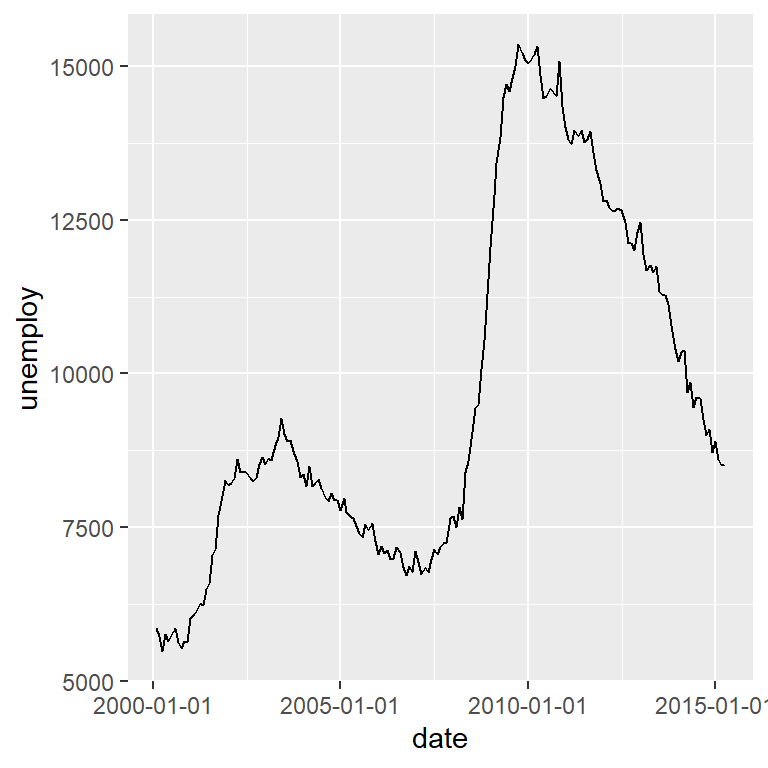Date format

The `date_labels` argument of the `scale_x_date` function can be used to customize the date format. In the following example we are setting `%Y-%m-%d`, which will show the year, the month and the day separated by hyphens instead of only the year.

``````# install.packages("ggplot2")
library(ggplot2)

# Data
df <- economics[economics\$date > as.Date("2000-01-01"), ]

ggplot(df, aes(x = date, y = unemploy)) +
geom_line() +
scale_x_date(date_labels = "%Y-%m-%d")``````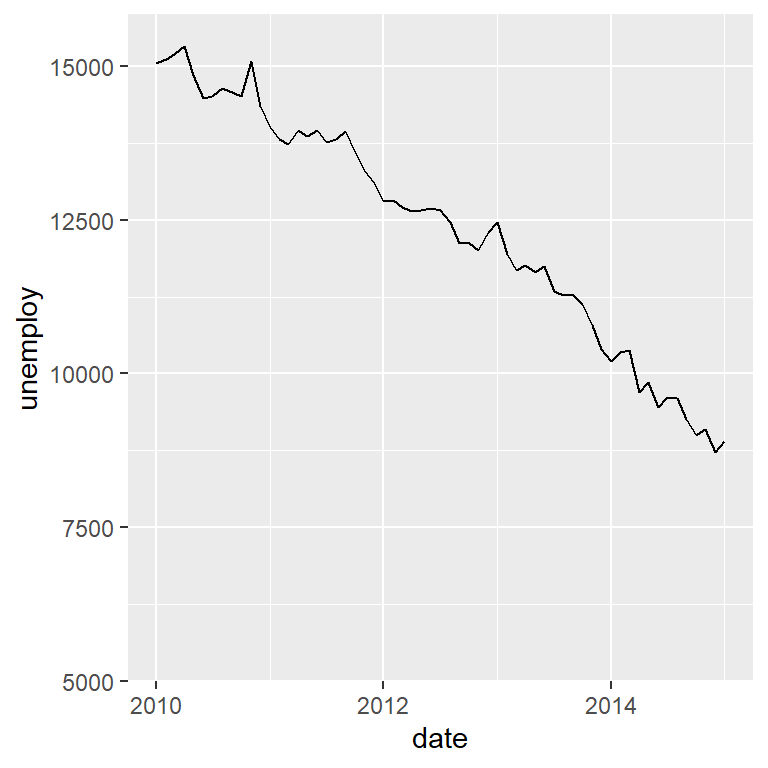Limits

You can set the limit of the axis based on dates with the `limit` argument of the function.

``````# install.packages("ggplot2")
library(ggplot2)

# Data
df <- economics[economics\$date > as.Date("2000-01-01"), ]

ggplot(df, aes(x = date, y = unemploy)) +
geom_line() +
scale_x_date(limit = c(as.Date("2010-01-01"), as.Date("2015-01-01")))``````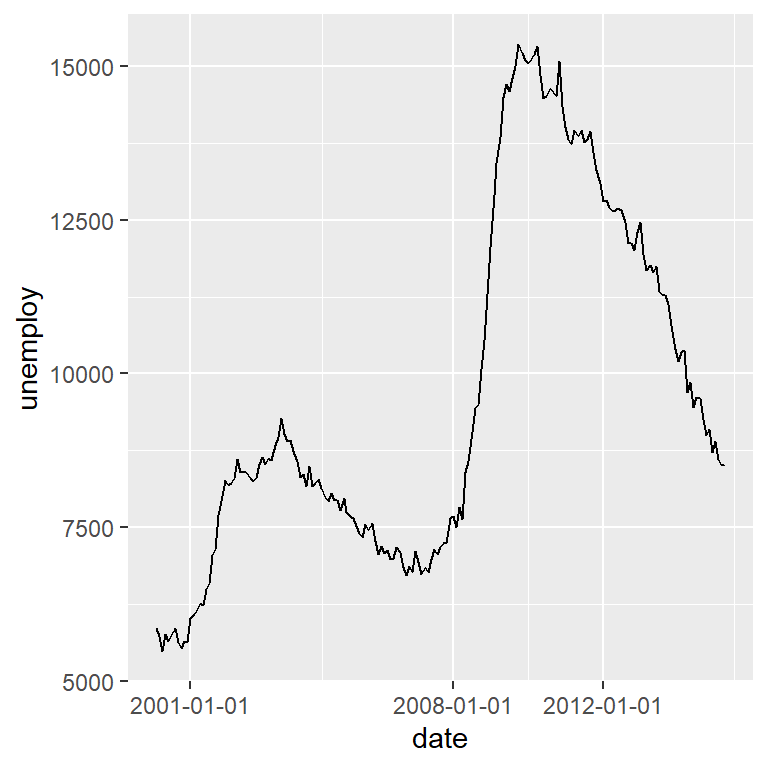Breaks

You can also specify the breaks with the `breaks` argument of the function, specifying the dates to be used as axis breaks.

``````# install.packages("ggplot2")
library(ggplot2)

# Data
df <- economics[economics\$date > as.Date("2000-01-01"), ]

ggplot(df, aes(x = date, y = unemploy)) +
geom_line() +
scale_x_date(breaks = c(as.Date("2001-01-01"),
as.Date("2008-01-01"),
as.Date("2012-01-01")))``````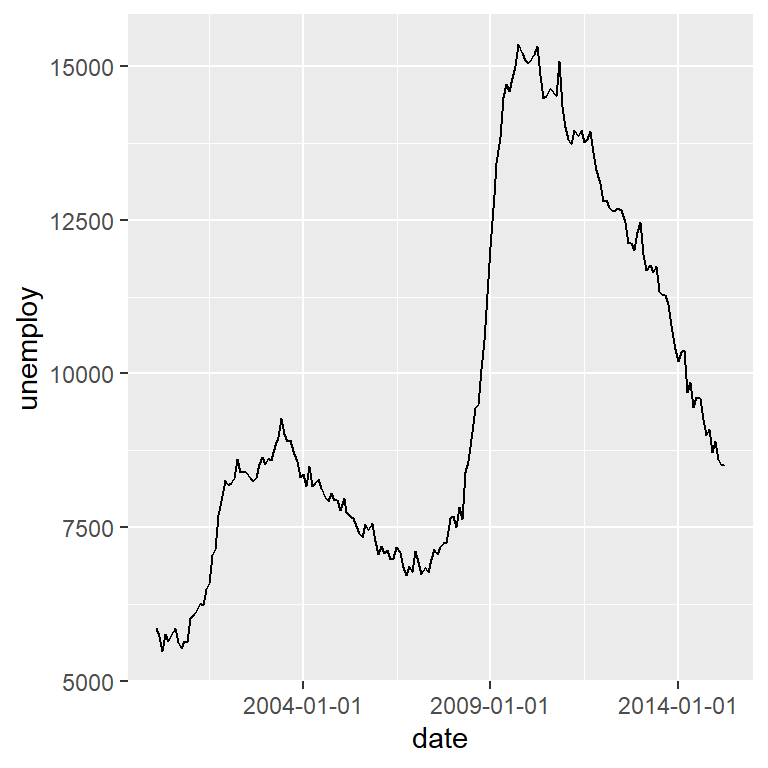An alternative is to specify the breaks with strings of the format `"1 day"` (a break each day), `"2 months"` (a break every two months), `"6 years"` (a break every six years), …

``````# install.packages("ggplot2")
library(ggplot2)

# Data
df <- economics[economics\$date > as.Date("2000-01-01"), ]

ggplot(df, aes(x = date, y = unemploy)) +
geom_line() +
scale_x_date(date_breaks = "5 years")``````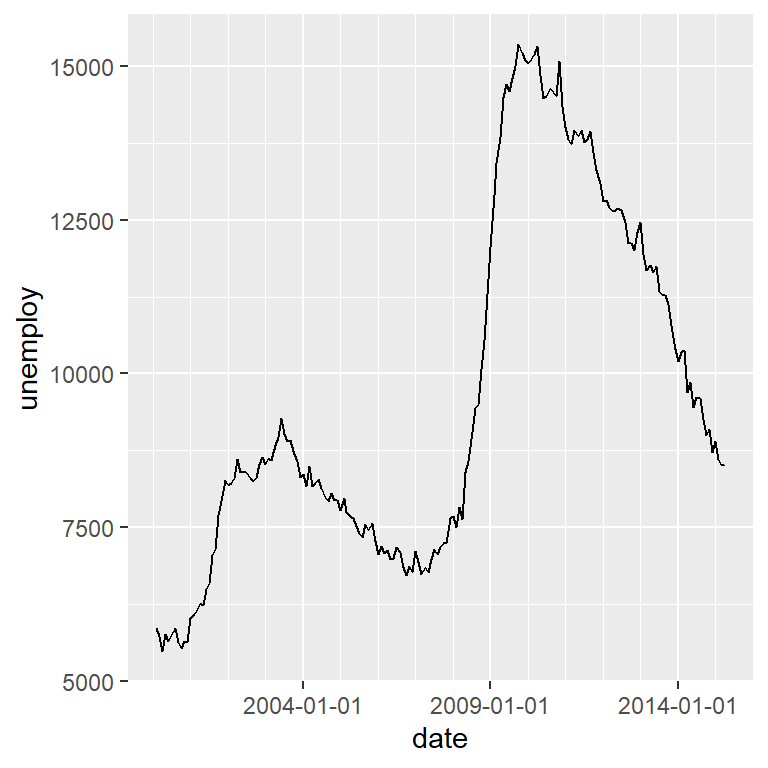The function also provides an argument named `date_minor_breaks` to set the minor breaks of the axis. This argument behaves the same as `date_breaks`.

``````# install.packages("ggplot2")
library(ggplot2)

df <- economics[economics\$date > as.Date("2000-01-01"), ]

ggplot(df, aes(x = date, y = unemploy)) +
geom_line() +
scale_x_date(date_breaks = "5 years",
date_minor_breaks = "1 year")``````

## Highlight changes, peaks and valleys

You can add lines to highlight breakpoints or structural changes with the `geom_vline` or `geom_hline` functions to add vertical or horizontal lines, respectively.

``````# install.packages("ggplot2")
# install.packages("ggpmisc")
library(ggplot2)
library(ggpmisc)

# Data
df <- economics[economics\$date > as.Date("2000-01-01"), ]

ggplot(df, aes(x = date, y = unemploy)) +
geom_line() +
geom_vline(xintercept = as.Date("2007-09-15"),
linetype = 2, color = 2, linewidth = 1)``````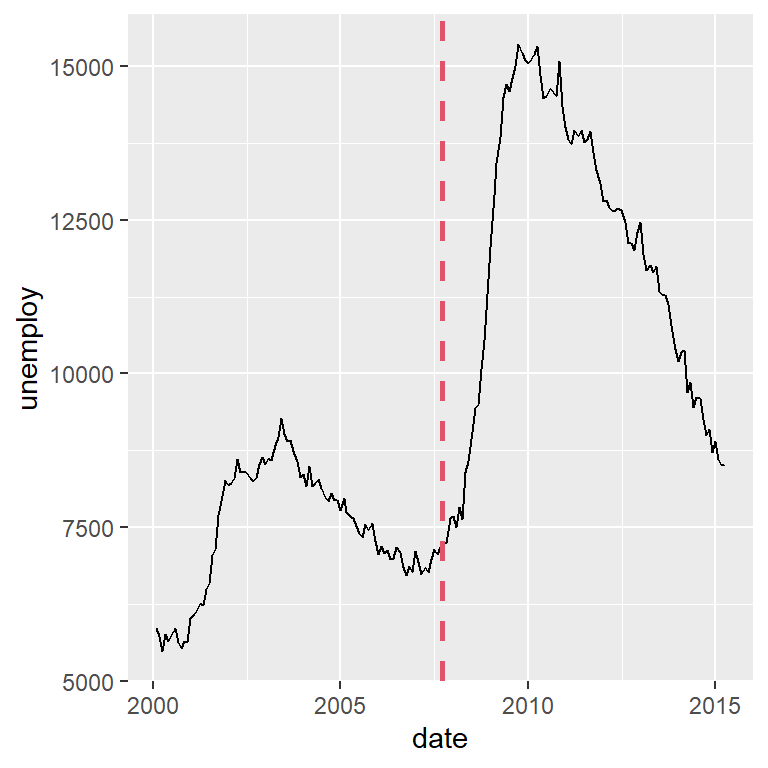Peaks and valleys

With the `stat_peaks` and `stat_valleys` functions from the `ggpmisc` package you can highlight local maximum or local minimum values based on a span (by default, `span = 5`). Both functions behave the same way and the geom used to represent the data can be passed to the `geom` argument, which defaults to `"point"`. In the following examples we add the peaks and valleys of the series, showing labels and a rug plot to display where the values are.

``````# install.packages("ggplot2")
# install.packages("ggpmisc")
library(ggplot2)
library(ggpmisc)

# Data
df <- economics[economics\$date > as.Date("2000-01-01"), ]

ggplot(df, aes(x = date, y = unemploy)) +
geom_line() +
stat_peaks(geom = "point", span = 15, color = "steelblue3", size = 2) +
stat_peaks(geom = "label", span = 15, color = "steelblue3", angle = 0,
hjust = -0.1, x.label.fmt = "%Y-%m-%d") +
stat_peaks(geom = "rug", span = 15, color = "blue", sides = "b")``````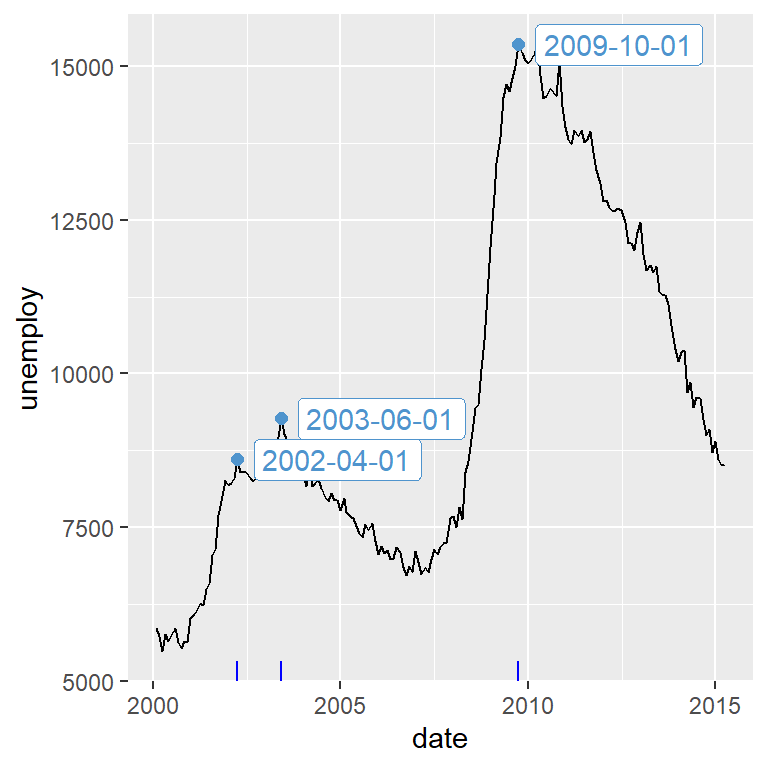``````# install.packages("ggplot2")
# install.packages("ggpmisc")
library(ggplot2)
library(ggpmisc)

# Data
df <- economics[economics\$date > as.Date("2000-01-01"), ]

ggplot(df, aes(x = date, y = unemploy)) +
geom_line() +
stat_valleys(geom = "point", span = 11, color = "red", size = 2) +
stat_valleys(geom = "label", span = 11, color = "red", angle = 0,
hjust = -0.1, x.label.fmt = "%Y-%m-%d") +
stat_valleys(geom = "rug", span = 11, color = "red", sides = "b")``````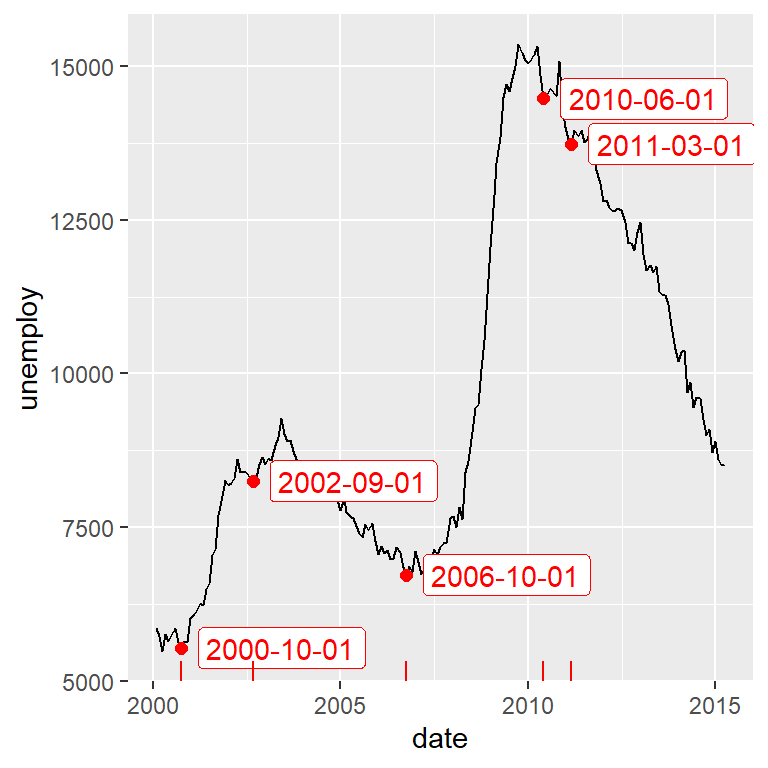With `geom_rect` it is possible to shade some areas of the time series. This is specially interesting to highlight trends, recessions, etc.

``````# install.packages("ggplot2")
# install.packages("ggpmisc")
library(ggplot2)
library(ggpmisc)

# Data
df <- economics[economics\$date > as.Date("2000-01-01"), ]

# Shade from 2000 to 2004 and from 2010 to 2015
shade <- data.frame(x1 = c(as.Date("2000-01-01"), as.Date("2010-01-01")),
x2 = c(as.Date("2004-01-01"), as.Date("2015-01-01")),
min = c(-Inf, -Inf), max = c(Inf, Inf))

ggplot() +
geom_line(data = df, aes(x = date, y = unemploy)) +
geom_rect(data = shade, aes(xmin = x1, xmax = x2, ymin = min, ymax = max),
fill = c("green", "red"), alpha = 0.2)``````